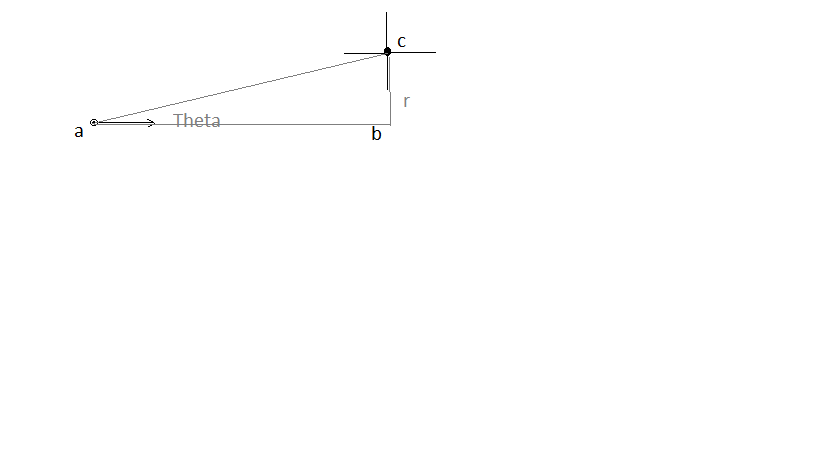# AP Physics C: Mechanics : Understanding Linear-Rotational Equivalents

## Example Questions

### Example Question #1 : Rotational Motion And Torque

What is the rotational equivalent of mass?

Moment of inertia

Angular momentum

Torque

Moment of inertia

Explanation:

The correct answer is moment of inertia. For linear equations, mass is what resists force and causes lower linear accelerations. Similarly, in rotational equations, moment of inertia resists torque and causes lower angular accelerations.

### Example Question #41 : Mechanics Exam

In rotational kinematics equations, what quantity is analogous to force in linear kinematics equations?

Torque

Moment of inertia

Impulse

Angular acceleration

Torque

Explanation:

Just as force causes linear acceleration, torque causes angular acceleration. This can be seen most in the linear-rotational comparison of Newton's second law: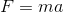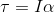### Example Question #49 : Mechanics Exam

A boot is put in a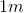stick which is attached to a rotor. The rotor turns with an angular velocity of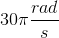. What is the linear velocity of the boot?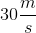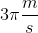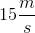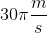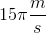Explanation:

Linear (tangential) velocity,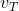is given by the following equation: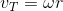Here,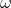is the angular velocity in radians per second andis the radius in meters.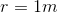Solve.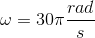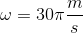### Example Question #1 : Rotational Motion And Torque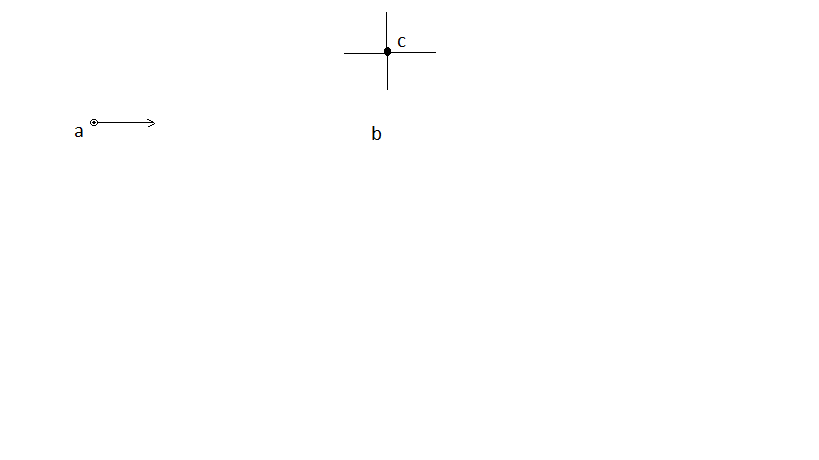A particle is moving at constant speed in a straight line past a fixed point in space, c. How does the angular momentum of the particle about the fixed point in space change as the particle moves from point a to point b?

It cannot be determined without knowing the mass of the particle

The angular momentum increases

The particle does not have angular momentum since it is not rotating

The angular momentum decreases

The angular momentum does not change

The angular momentum of a particle about a fixed axis is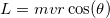. As the particle draws nearer the fixed axis, bothand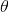change. However, the product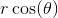remains constant. If you imagine a triangle connecting the three points, the productrepresents the"of closest approach", labeled "" in the diagram.